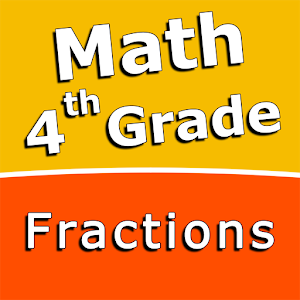# Fourth grade Math skills – FractionsRating: 5/5

Intuitive interface powered by handwriting input and a choice of three fun and engaging mini-games in addition to a regular math trainer mode makes our app stand out from the crowd of generic math learning apps.

With Fourth grade Math skills – Fractions you can practice and improve the following math skills:
– Add fractions with like denominators
– Subtract fractions with like denominators
– Add mixed numbers with like denominators
– Subtract mixed numbers with like denominators
– Add fractions with unlike denominators
– Subtract fractions with unlike denominators
– Add fractions with denominators of 10 and 100
– Add mixed numbers with unlike denominators
– Subtract mixed numbers with unlike denominators
– Multiply fractions by one-digit whole numbers

Choose between four different modes:
– Math Trainer
– Math Shot mini-game
– Math Gravity mini-game
– Math Trick Shot mini-game